Qucs-core  0.0.19
rectline Class Reference

#include <rectline.h>

Inheritance diagram for rectline: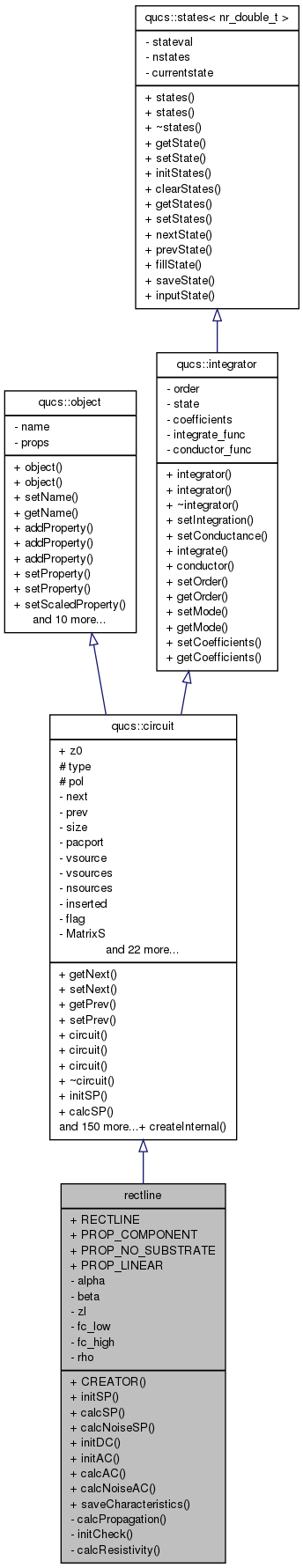[legend]
Collaboration diagram for rectline: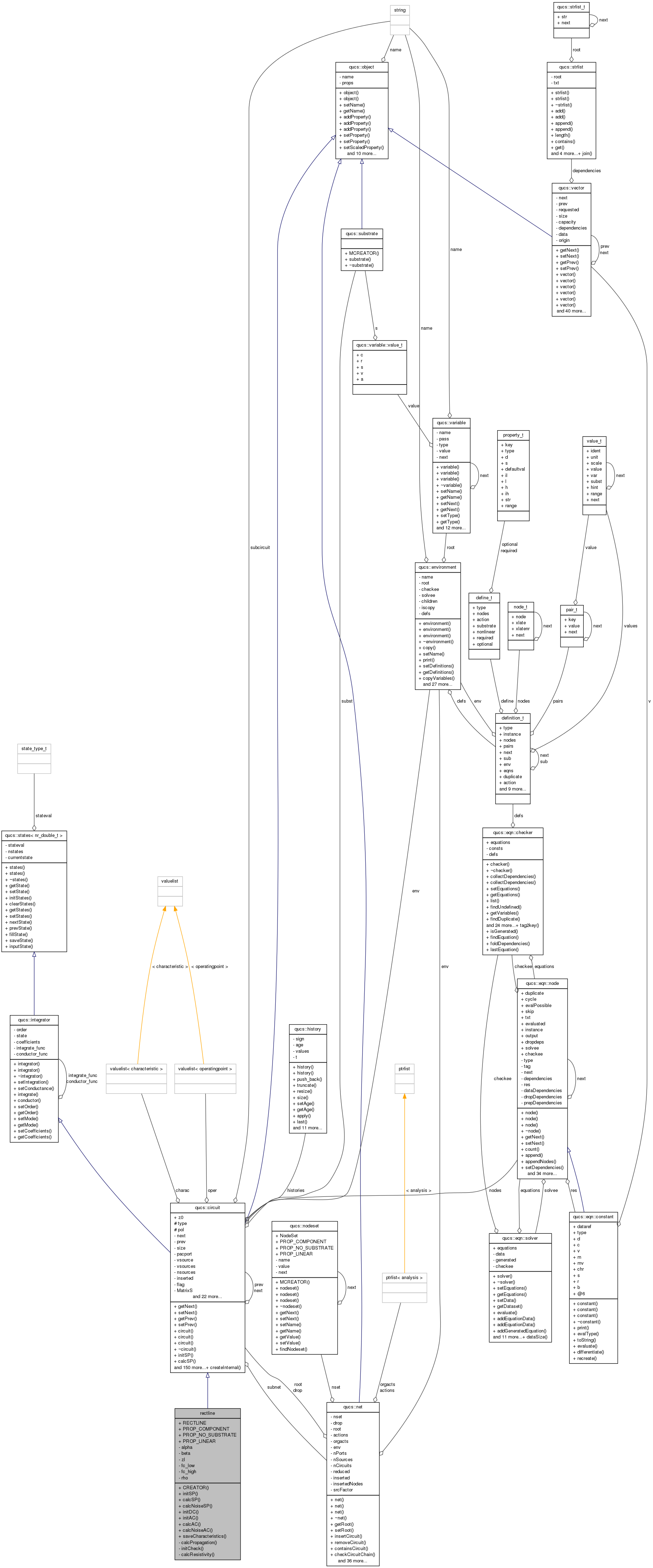[legend]

Public Member Functions

CREATOR (rectline)
void initSP (void)
void calcSP (nr_double_t)
void calcNoiseSP (nr_double_t)
void initDC (void)
void initAC (void)
void calcAC (nr_double_t)
void calcNoiseAC (nr_double_t)
void saveCharacteristics (nr_complex_t)

Data Fields

RECTLINE
PROP_COMPONENT
PROP_NO_SUBSTRATE
PROP_LINEAR

Private Member Functions

void calcPropagation (nr_double_t)
void initCheck (void)
void calcResistivity (const char *const, nr_double_t)

Private Attributes

nr_double_t alpha
nr_double_t beta
nr_complex_t zl
nr_double_t fc_low
nr_double_t fc_high
nr_double_t rho

Detailed Description

A TE10 rectangular waveguide component

Note:
Evanecent mode are not implemented
Todo:
Implement evanecent mode

Definition at line 318 of file rectline.cpp.

Member Function Documentation

 void rectline::calcAC ( nr_double_t frequency )  [virtual]

calc propagation using classical transmission line formulae

Reimplemented from qucs::circuit.

Definition at line 280 of file rectline.cpp.

 void rectline::calcNoiseAC ( nr_double_t )  [virtual]

Compute noise

Reimplemented from qucs::circuit.

Definition at line 295 of file rectline.cpp.

 void rectline::calcNoiseSP ( nr_double_t )  [virtual]

Compute noise parameter

Reimplemented from qucs::circuit.

Definition at line 202 of file rectline.cpp.

 void rectline::calcPropagation ( nr_double_t frequency )  [private]

Compute propagation constant

According to  table 3.2 p 128 Wawe number in vacuum is: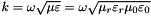Where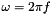,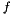is the frequency,  the magnetic permeability,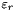the permitivity. Using well known formula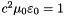, we found: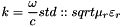In general caseis: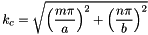Where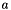is the wider dimension of the guide and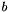the smaller and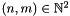. In the TE10 case it simplifies to: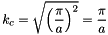The propagation constant is: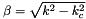Loss could be divised in dielectric and resistive loss. Dielectric loss are computed using: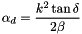Resistive using  eq (3.96) p 125 (valid only for TE10 mode)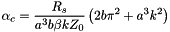Wave impedance is for TE10: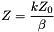Definition at line 153 of file rectline.cpp.

 void rectline::calcResistivity ( const char * const Mat, nr_double_t T )  [private]

Definition at line 63 of file rectline.cpp.

 void rectline::calcSP ( nr_double_t frequency )  [virtual]

Compute S parameter use generic transmission line formulae

Reimplemented from qucs::circuit.

Definition at line 246 of file rectline.cpp.

 rectline::CREATOR ( rectline )
 void rectline::initAC ( void )  [virtual]

init AC

Reimplemented from qucs::circuit.

Definition at line 273 of file rectline.cpp.

 void rectline::initCheck ( void )  [private]

Check validity of parameter and compute cutoff frequencies

Note:
do not check validity of epsr or mur because some research stuff could use epsr < 1 (plasma)

Definition at line 215 of file rectline.cpp.

 void rectline::initDC ( void )  [virtual]

Reimplemented from qucs::circuit.

Definition at line 266 of file rectline.cpp.

 void rectline::initSP ( void )  [virtual]

initialize S-parameters

Reimplemented from qucs::circuit.

Definition at line 239 of file rectline.cpp.

 void rectline::saveCharacteristics ( nr_complex_t )  [virtual]

Reimplemented from qucs::circuit.

Definition at line 234 of file rectline.cpp.

Field Documentation

 nr_double_t rectline::alpha [private]

attenuation constant

Definition at line 49 of file rectline.h.

 nr_double_t rectline::beta [private]

propagation constant

Definition at line 51 of file rectline.h.

 nr_double_t rectline::fc_high [private]

cut off frequency higher mode

Definition at line 57 of file rectline.h.

 nr_double_t rectline::fc_low [private]

cut off frequency lower bound

Definition at line 55 of file rectline.h.

Definition at line 319 of file rectline.cpp.

Definition at line 319 of file rectline.cpp.

Definition at line 319 of file rectline.cpp.

Definition at line 319 of file rectline.cpp.

 nr_double_t rectline::rho [private]

resistivity

Definition at line 59 of file rectline.h.

 nr_complex_t rectline::zl [private]

wave impedance

Definition at line 53 of file rectline.h.

The documentation for this class was generated from the following files: